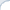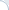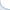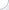UPDATE: BM25 implementation has changed in recent Lucene versions. For more details, see the post Accurate Lucene BM25 : Redux.

In this blog post, I explain why Lucene's BM25 implementation is not accurate and propose its efficient replacement. I will also cover the following three little-known topics related to BM25 and sometimes other similarity models:

1. Lossy document length encoding in Lucene indexing;
2. An arcane method of index-time boosting (and why you probably don't want to use it);
3. An omission in Lucene's indexing tutorial related to choosing the right similarity during indexing.

The efficiency and effectiveness of my BM25 replacement is verified using two collections created from community question-answering data. One collection is publicly available so that my experiments can be easily reproduced by people without academic affiliations (see code in my GitHub repo). I think this should be especially interesting to researchers using Lucene's BM25 as a baseline.

Among other similarity models, Lucene employs the BM25 similarity. It is a variant of the TF*IDF scheme, where the normalized term frequency (i.e., TF) is computed using the following formula:

$$\frac{ \text{freq} \cdot (k_1 + 1) } { \text{freq} + k_1 \cdot \left(1 - b + b \cdot |D| \cdot \text{iboost}^{-2} \cdot |D|^{-1}_{\text{avg}} \right) }, \textbf{(*)}$$

where freq is a raw, i.e., unnormalized term frequency, $|D|$ is a document length in words, $|D|_{\text{avg}}$ is an average document length, and iboost is an index-time boosting factor ($k_1$ and $b$ are parameters).

First, let us talk about the boosting factor. It works by reducing the document length and, consequently, the denominator in the equation (*). Hence, increase in iboost leads to increase in the normalized term frequency and, thus, in the overall score. However, the relationship between the index-time boosting factor is quite convoluted and I really doubt that such a boosting scheme is usable in practice.

One may wonder why the index-time boosting is implemented in such an unusual fashion as opposed to introducing a simple multiplicative factor. The reason is that Lucene's API does not support such multiplicative factors directly. Therefore, a developer of a BM25 similarity class had to bundle index-time boosting with computation of the document-length normalization factor. As we read in the documentation for the latest Lucene version: " At indexing time, the indexer calls computeNorm(FieldInvertState), allowing the Similarity implementation to set a per-document value for the field that will be later accessible via LeafReader.getNormValues(String). Lucene makes no assumption about what is in this norm, but it is most useful for encoding length normalization information. "

It is the hook function computeNorm(FieldInvertState) that computes the value $|D| \cdot \text{iboost}^{-2}$ and compresses it into a one-byte value. Because of this lossy compression, there are only 256 possible normalization factors. Therefore, we can precompute the value of $k_1 \cdot \left(1 - b + b \cdot |D| \cdot \text{iboost}^{-2} \cdot |D|^{-1}_{\text{avg}} \right)$ that participates in Eq. (*) and avoid recomputation during query-time.

This memoization technique seems to result in a noticeable speed up, but it also substantially degrades the quality of Lucene's BM25 ranking (due to the lossy normalization compression). In what follows, I will describe experiments where (depending on the effectiveness metric and data type) performance loss is 5-10%. The collections that I use are rather small: 4-6 million short documents. I suspect that the degradation becomes more noticeable as the collection size increases. This may not matter in all applications, of course, but it is quite aggravating if you use Lucene BM25 as one of the baselines in your experiments.

Before I proceed with the experiments, I want to highlight that document length normalization factors may be (and mostly are) incompatible among different similarities. For this reason, one need to use exactly the same similarity during both indexing (see, e.g., my code here) and retrieval. This fact, however, seems to be missing from the Lucene's demo/tutorial file. If you use Lucene 6 and BM25, this will not be a problem, because BM25 is now the default similarity (and BM25 parameters are not used during the computation of the document length normalization). Yet, this would be a problem in Lucene 4 or 5, where the default similarity is different from BM25. Likewise, if you implement a custom similarity class, you may need to specify it both during indexing and retrieval.

For the purpose of experiments, I use two community question answering (QA) data sets: Yahoo! Answers collection L6 (shortly Comprehensive) and Stack Overflow (code excluded). These collections are used to assess effectiveness and efficiency of two BM25 implementation for Lucene. The first implementation is the standard BM25Similarity in Lucene 6. The second implementation (class BM25SimilarityFix) is a modification of Lucene's similarity class. This modification does not use an approximation for the document length.

Community QA data allows us to test the quality of retrieval algorithms by measuring the accuracy at the task of retrieving answers by using respective questions as queries. While the overall effectiveness of such method may not be good enough to be useful in practice, we can experiment with large collections of queries without the need to manually annotate thousands (or millions) retrieval results as it is done, e.g., in the TREC evaluation.

More specifically, I first retrieve 100 most highly ranked documents and compare effectiveness using several standard IR metrics: the precision/accuracy at rank one (P@1), the recall at rank 10 (Recall@10), the mean average precision (MAP) and the overall answer recall (which is technically Recall@100). A disadvantage of the community QA data is that there may be more than one relevant answer when users submit similar questions. Given a question, relevant best answers posted for similar questions might be even more relevant than the best answer posted for this question. I personally think that such outcomes should be quite infrequent, but I do not have good numbers to back up my hypothesis. In any case, we will keep in mind that the accuracy at rank 1 (P@1) and the mean reciprocal rank might be slightly biased. However, I do not see a good reason why a better system should not find respective best answers more frequently, in particular, among top 10 highest ranked results. In other words, I think we can pretty much trust metrics such as recall at rank 10 (Recall@10).

The above described collections are used to assess effectiveness and efficiency of two BM25 implementations. To this end, I search using the same set of 10 thousand queries 11 times (each set of queries uses 10 thousands first questions from a collection). The hardware is Intel(R) Xeon(R) CPU E5-1410 @ 2.80GHz with 10 MB of cache and 32 GB of RAM. The tests are run on Linux using Java 8. The first retrieval run is used to "warm up" the index. The results for the following 10 runs are used to compute efficiency.

Most evaluation work is done by the script run_eval_queries, which also computes p-values using the two-sided paired t-test (for this you need R and Python). Before doing this, of course, you would need to create a Lucene index. A more detailed description of the experimental process is given in the README file.

Retrieval time
Average
(ms)
Retrieval time
SD (ms)
P@1 Recall@10 MAP Recall@100
Lucene BM25 38.2 3.5 0.0722 0.1666 0.1043 0.2925
Accurate BM25 39.5 1.3 0.0768 0.1742 0.1098 0.2969
Gain 3.4% 6.4% 4.6% 5.2% 1.5%
p-value 9E-05 6E-08 1E-12 0.0015
Stack Overflow
Lucene BM25 338.2 24.2 0.065 0.1494 0.0937 0.2927
Accurate BM25 356.4 19.2 0.0712 0.1588 0.1009 0.3037
Gain 5.4% 9.5% 6.3% 7.7% 3.8%
p-value 1E-06 5E-09 2E-16 2E-09

The experimental results are given in the table. First, we can see that the standard Lucene's implementation is, indeed, a tad faster: by 3% for Comprehensive and by 5% for StackOverflow (for a more reliable comparison, though, I should have carried out more experiments, because currently these differences are within one standard deviation from respective means). At the same, the standard implementation is substantially less effective. In particular, for Comprehensive it is 6.4% worse in P@1 and 4.6% worse in Recall@10. These substantial differences are also statistically significant (the statistically significance tests generate tiny p-values).

The difference is smaller if we consider recall at rank 100. This is not especially surprising, because our collections are relatively small (4.4M and 6.2M indexed answers). So, in many cases Lucene is able to find relevant answers, but it cannot rank them high enough using the current implementation of BM25. My guess is that the gap between implementations would increase if many more answers were indexed.

I am not going to speculate whether a 3-5% loss in efficiency is worth a 5-10% gain in accuracy. Ultimately, the user would decide. However, if you employ Lucene BM25 as a baseline in IR experiments, you should probably not use the standard Lucene BM25 similarity due to the potential loss in accuracy. Of course, I may be wrong. So, I encourage the readers to scrutinize my code.

To conclude, I would note that it would be possible to further optimize the existing similarity (while keeping it accurate), if we could recompute normalization factors after the collection is created. Specifically, we could have precomputed the value $k_1 \cdot \left(1 - b + b \cdot |D| \cdot \text{iboost}^{-2} \cdot |D|^{-1}_{\text{avg}} \right)$ that participates in Eq. (*) for each document (technically, we would also need to store the float in the long-type format, however, it is possible by converting a float via floatToRawIntBits). However, such an precomputation is not possible with the current API.